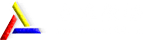### 金准数据 GoogleBrain新技术：No RNNs, No CNNs, Just Attention！

• 11个月前发布
• 发布人：管理员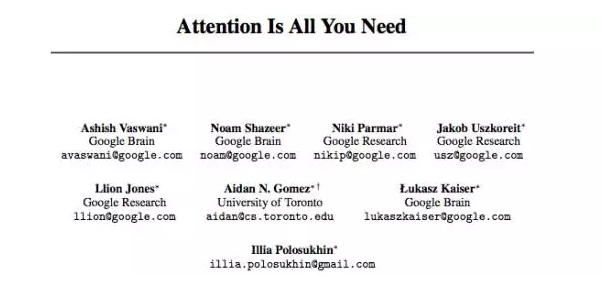1 Introduction

Recurrent neural networks, long short-term memory  and gated recurrent  neural networks in particular, have been firmly established as state of the art approaches in sequence modeling and transduction problems such as language modeling and machine translation [31, 2, 5]. Numerous efforts have since continued to push the boundaries of recurrent language models and encoder-decoder architectures [34, 22, 14].

Recurrent models typically factor computation along the symbol positions of the input and output sequences. Aligning the positions to steps in computation time, they generate a sequence of hidden states ht, as a function of the previous hidden state ht−1 and the input for position t. This inherently sequential nature precludes parallelization within training examples, which becomes critical at longer sequence lengths, as memory constraints limit batching across examples. Recent work has achieved significant improvements in computational efficiency through factorization tricks  and conditional ∗Equal contribution. Listing order is random. †Work performed while at Google Brain. arXiv:1706.03762v1 [cs.CL] 12 Jun 2017 computation , while also improving model performance in case of the latter. The fundamental constraint of sequential computation, however, remains.

Attention mechanisms have become an integral part of compelling sequence modeling and transduction models in various tasks, allowing modeling of dependencies without regard to their distance in the input or output sequences [2, 17]. In all but a few cases , however, such attention mechanisms are used in conjunction with a recurrent network.

In this work we propose the Transformer, a model architecture eschewing recurrence and instead relying entirely on an attention mechanism to draw global dependencies between input and output. The Transformer allows for significantly more parallelization and can reach a new state of the art in translation quality after being trained for as little as twelve hours on eight P100 GPUs.

2 Background

The goal of reducing sequential computation also forms the foundation of the Extended Neural GPU , ByteNet  and ConvS2S , all of which use convolutional neural networks as basic building block, computing hidden representations in parallel for all input and output positions. In these models, the number of operations required to relate signals from two arbitrary input or output positions grows in the distance between positions, linearly for ConvS2S and logarithmically for ByteNet. This makes it more difficult to learn dependencies between distant positions . In the Transformer this is reduced to a constant number of operations, albeit at the cost of reduced effective resolution due to averaging attention-weighted positions, an effect we counteract with Multi-Head Attention as described in section 3.2.

Self-attention, sometimes called intra-attention is an attention mechanism relating different positions of a single sequence in order to compute a representation of the sequence. Self-attention has been used successfully in a variety of tasks including reading comprehension, abstractive summarization, textual entailment and learning task-independent sentence representations [4, 25, 26, 20].

To the best of our knowledge, however, the Transformer is the first transduction model relying entirely on self-attention to compute representations of its input and output without using RNNs or convolution. In the following sections, we will describe the Transformer, motivate self-attention and discuss its advantages over models such as [15, 16] and .

3 Model Architecture

Most competitive neural sequence transduction models have an encoder-decoder structure [5, 2, 31]. Here, the encoder maps an input sequence of symbol representations (x1, ..., xn) to a sequence of continuous representations z = (z1, ..., zn). Given z, the decoder then generates an output sequence (y1, ..., ym) of symbols one element at a time. At each step the model is auto-regressive , consuming the previously generated symbols as additional input when generating the next.

The Transformer follows this overall architecture using stacked self-attention and point-wise, fully connected layers for both the encoder and decoder, shown in the left and right halves of Figure 1, respectively.

3.1 Encoder and Decoder Stacks

Encoder: The encoder is composed of a stack of N = 6 identical layers. Each layer has two sub-layers. The first is a multi-head self-attention mechanism, and the second is a simple, positionwise fully connected feed-forward network. We employ a residual connection around each of the two sub-layers, followed by layer normalization . That is, the output of each sub-layer is LayerNorm(x + Sublayer(x)), where Sublayer(x) is the function implemented by the sub-layer itself. To facilitate these residual connections, all sub-layers in the model, as well as the embedding layers, produce outputs of dimension dmodel = 512.

Decoder: The decoder is also composed of a stack of N = 6 identical layers. In addition to the two sub-layers in each encoder layer, the decoder inserts a third sub-layer, which performs multi-head attention over the output of the encoder stack. Similar to the encoder, we employ residual connectionsFigure 1: The Transformer - model architecture. around each of the sub-layers, followed by layer normalization. We also modify the self-attention sub-layer in the decoder stack to prevent positions from attending to subsequent positions. This masking, combined with fact that the output embeddings are offset by one position, ensures that the predictions for position i can depend only on the known outputs at positions less than i.

3.2 Attention

An attention function can be described as mapping a query and a set of key-value pairs to an output, where the query, keys, values, and output are all vectors. The output is computed as a weighted sum of the values, where the weight assigned to each value is computed by a compatibility function of the query with the corresponding key.

3.2.1 Scaled Dot-Product Attention

We call our particular attention "Scaled Dot-Product Attention" (Figure 2). The input consists of queries and keys of dimension dk, and values of dimension dv. We compute the dot products of the query with all keys, divide each by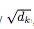, and apply a softmax function to obtain the weights on the values.

In practice, we compute the attention function on a set of queries simultaneously, packed together into a matrix Q. The keys and values are also packed together into matrices K and V . We compute the matrix of outputs as: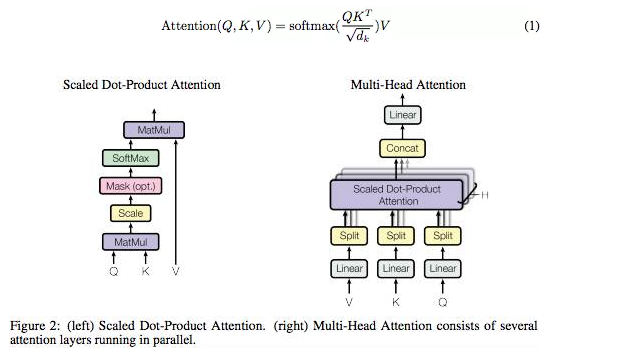The two most commonly used attention functions are additive attention , and dot-product (multiplicative) attention. Dot-product attention is identical to our algorithm, except for the scaling factor of. Additive attention computes the compatibility function using a feed-forward network with a single hidden layer. While the two are similar in theoretical complexity, dot-product attention is much faster and more space-efficient in practice, since it can be implemented using highly optimized matrix multiplication code. While for small values of dk the two mechanisms perform similarly, additive attention outperforms dot product attention without scaling for larger values of dk . We suspect this to be caused by the dot products growing too large in magnitude to result in useful gradients after applying the softmax function. To counteract this, we scale the dot product by 1/Instead of performing a single attention function with dmodel-dimensional keys, values and queries, we found it beneficial to linearly project the queries, keys and values h times with different, learned linear projections to dk, dk and dv dimensions, respectively. On each of these projected versions of queries, keys and values we then perform the attention function in parallel, yielding dv-dimensional output values. These are concatenated, resulting in the final values, as depicted in Figure 2 Multi-head attention allows the model to jointly attend to information from different representation subspaces at different positions. With a single attention head, averaging inhibits this.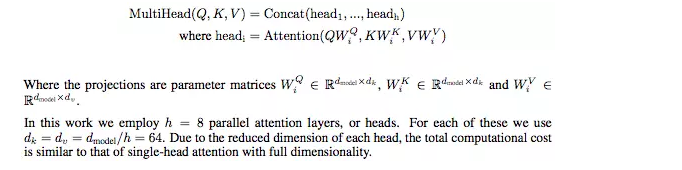3.2.3 Applications of Attention in our Model

The Transformer uses multi-head attention in three different ways:

• In the encoder-decoder attention layers, the queries come from the previous decoder layer, and the memory keys and values come from the output of the encoder. This allows every 4 position in the decoder to attend over all positions in the input sequence. This mimics the typical encoder-decoder attention mechanisms in sequence-to-sequence models such as [34, 2, 9].

• The encoder contains self-attention layers. In a self-attention layer all of the keys, values and queries come from the same place, in this case, the output of the previous layer in the encoder. Each position in the encoder can attend to all positions in the previous layer of the encoder.

• Similarly, self-attention layers in the decoder allow each position in the decoder to attend to all positions in the decoder up to and including that position. We need to prevent leftward information flow in the decoder to preserve the auto-regressive property. We implement this inside of scaled dot-product attention by masking out (setting to −∞) all values in the input of the softmax which correspond to illegal connections. See Figure 2.

3.3 Position-wise Feed-Forward Networks

In addition to attention sub-layers, each of the layers in our encoder and decoder contains a fully connected feed-forward network, which is applied to each position separately and identically. This consists of two linear transformations with a ReLU activation in between.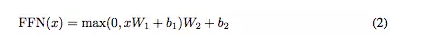While the linear transformations are the same across different positions, they use different parameters from layer to layer. The dimension of input and output is dmodel, and the inner-layer has dimension 2048.

3.4 Embeddings and Softmax

Similarly to other sequence transduction models, we use learned embeddings to convert the input tokens and output tokens to vectors of dimension dmodel. We also use the usual learned linear transformation and softmax function to convert the decoder output to predicted next-token probabilities. In our model, we share the same weight matrix between the two embedding layers and the pre-softmax linear transformation. In the embedding layers, we multiply those weights by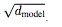3.5 Positional Encoding

Since our model contains no recurrence and no convolution, in order for the model to make use of the order of the sequence, we must inject some information about the relative or absolute position of the tokens in the sequence. To this end, we add "positional encodings" to the input embeddings at the bottoms of the encoder and decoder stacks. The positional encodings have the same dimension dmodel as the embeddings, so that the two can be summed. There are many choices of positional encodings, learned and fixed . In this work, we use sine and cosine functions of different frequencies:where pos is the position and i is the dimension. That is, each dimension of the positional encoding corresponds to a sinusoid. The wavelengths form a geometric progression from 2π to 10000 · 2π. We chose this function because we hypothesized it would allow the model to easily learn to attend by relative positions, since for any fixed offset k,can be represented as a linear function of P Epos.

4 Why Self-Attention

In this section we compare various aspects of self-attention layers to the recurrent and convolutional layers commonly used for mapping one variable-length sequence of symbol representations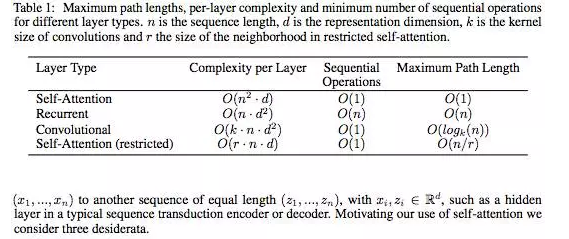One is the total computational complexity per layer. Another is the amount of computation that can be parallelized, as measured by the minimum number of sequential operations required.

The third is the path length between long-range dependencies in the network. Learning long-range dependencies is a key challenge in many sequence transduction tasks. One key factor affecting the ability to learn such dependencies is the length of the paths forward and backward signals have to traverse in the network. The shorter these paths between any combination of positions in the input and output sequences, the easier it is to learn long-range dependencies . Hence we also compare the maximum path length between any two input and output positions in networks composed of the different layer types.

As noted in Table 1, a self-attention layer connects all positions with a constant number of sequentially executed operations, whereas a recurrent layer requires O(n) sequential operations. In terms of computational complexity, self-attention layers are faster than recurrent layers when the sequence length n is smaller than the representation dimensionality d, which is most often the case with sentence representations used by state-of-the-art models in machine translations, such as word-piece  and byte-pair  representations. To improve computational performance for tasks involving very long sequences, self-attention could be restricted to considering only a neighborhood of size r in the input sequence centered around the respective output position. This would increase the maximum path length to O(n/r). We plan to investigate this approach further in future work.

A single convolutional layer with kernel width k < n does not connect all pairs of input and output positions. Doing so requires a stack of O(n/k) convolutional layers in the case of contiguous kernels, or O(logk(n)) in the case of dilated convolutions , increasing the length of the longest paths between any two positions in the network. Convolutional layers are generally more expensive than recurrent layers, by a factor of k. Separable convolutions , however, decrease the complexity considerably, to. Even with k = n, however, the complexity of a separable convolution is equal to the combination of a self-attention layer and a point-wise feed-forward layer, the approach we take in our model.

As side benefit, self-attention could yield more interpretable models. We inspect attention distributions from our models and present and discuss examples in the appendix. Not only do individual attention heads clearly learn to perform different tasks, many appear to exhibit behavior related to the syntactic and semantic structure of the sentences.

5 Training

This section describes the training regime for our models.

5.1 Training Data and Batching

We trained on the standard WMT 2014 English-German dataset consisting of about 4.5 million sentence pairs. Sentences were encoded using byte-pair encoding , which has a shared sourcetarget vocabulary of about 37000 tokens. For English-French, we used the significantly larger WMT 6 2014 English-French dataset consisting of 36M sentences and split tokens into a 32000 word-piece vocabulary . Sentence pairs were batched together by approximate sequence length. Each training batch contained a set of sentence pairs containing approximately 25000 source tokens and 25000 target tokens.

5.2 Hardware and Schedule

We trained our models on one machine with 8 NVIDIA P100 GPUs. For our base models using the hyperparameters described throughout the paper, each training step took about 0.4 seconds. We trained the base models for a total of 100,000 steps or 12 hours. For our big models,(described on the bottom line of table 3), step time was 1.0 seconds, and the models were trained for 300,000 steps or 3.5 days.

5.3 OptimizerThis corresponds to increasing the learning rate linearly for the first warmup_steps training steps, and decreasing it thereafter proportionally to the inverse square root of the step number. We used warmup_steps = 4000.

5.4 Regularization

We employ three types of regularization during training: Residual Dropout We apply dropout  to the output of each sub-layer, before it is added to the sub-layer input and normalized. In addition, we apply dropout to the sums of the embeddings and the positional encodings in both the encoder and decoder stacks. For the base model, we use a rate of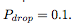Attention Dropout Query to key attentions are structurally similar to hidden-to-hidden weights in a feed-forward network, albeit across positions. The softmax activations yielding attention weights can then be seen as the analogue of hidden layer activations. A natural possibility is to extend dropout  to attention. We implement attention dropout by dropping out attention weights as,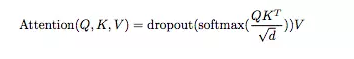In addition to residual dropout, we found attention dropout to be beneficial for our parsing experiments.

Label Smoothing During training, we employed label smoothing of value ls = 0.1 . This hurts perplexity, as the model learns to be more unsure, but improves accuracy and BLEU score.

6 Results

6.1 Machine Translation

On the WMT 2014 English-to-German translation task, Our big transformer model (Transformer (big) in Table 2) outperforms the best previously reported models (including ensembles) by more than 2.0 BLEU, establishing a new state-of-the-art BLEU score of 28.4. The configuration of this model is listed in the bottom line of Table 3. Training took 3.5 days on 8 P100 GPUs. Even our base model surpasses all previously published models and ensembles, at a fraction of the training cost of any of the previous best models.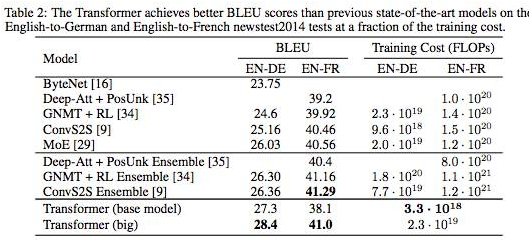On the WMT 2014 English-to-French translation task, our big model achieves a BLEU score of 41.0, outperforming all of the previously published single models, at less than 1/4 the training cost of the previous state-of-the-art model. The Transformer (big) model trained for English-to-French used dropout rate Pdrop = 0.1, instead of 0.3.

For the ba

##### 印度是如何一步步蚕食并在最后吞并锡金的？

43年前的今天，1975年4月10日，锡金议会通过决议废黜国王，要求把锡金并入印度。锡金原为独立的世袭君主国，1972年结束封建制度...

##### 伯克希尔现金储备升至1280亿美元 巴菲特仍持币观望

伯克希尔哈撒韦(323400,4461.00,1.40%)公司（BerkshireHathaway）的巨额现金储备仍在不断扩大，但沃伦-巴菲特（WarrenBuffet...